# Order of Operations (PEMDAS)

We perform different operations in mathematics to solve problems related to our everyday life. Some common operations we do regularly in arithmetic are addition, subtraction, multiplication, division, and squaring.

Order of operations describes how we perform operations in an expression. Let us consider the given expression with integers:

6 × (3 + 62 – 5) ÷ 5

Which part should we calculate first?

We need to follow the order of operation or operator precedence rule known as PEMDAS when we go from left to right of an expression.

## What are the Orders of Operation

The mathematical orders of operation are the rules describing the sequence in which the operations in an expression are performed.

It was invented in 1913 by Webster Wells and Walter W. Hart.

How Do I Remember It

The way to remember the order of operations is PEMDAS. The steps are:

### Step 1: P for Parentheses

The first is to solve all the operations within parenthesis ( ), { }, [ ]. All the expressions within the parenthesis are considered a group.

### Step 2: E for Exponent

The next step is solving the expressions with an exponent (powers and exponents).

### Step 3: MD for Multiplication and Division

These two operations (×) and (÷) rank equally and should be performed in the third step. Do the operation that comes first.

### Step 4: AS for Addition and Subtraction

Finally, similar to multiplication and division, addition (+) and subtraction (-) also rank equally and should be performed next to them.

Some other mnemonics are:

• Please Eat Mom’s Delicious Apple Strudels
• Popcorn Every Monday Donuts Always Sunday

In the United Kingdom, they apply BODMAS instead of PEMDAS.

B for brackets, O for orders, D for divide, M for multiply, A for add, and S for subtract

B for bracket, E for exponent, D for divide, M for multiply, A for add, and S for subtract

Now let us solve the expression given to us at the start of this article: 6 × (3 + 62 – 5) ÷ 5 using PEMDAS.

Here in the expression 6 × (3 + 62 – 5) ÷ 5

(3 + 62 – 5) is within parenthesis and should be performed first. However, there are two separate operations + and – . By the PEMDAS rule, exponent 62 should be done first. Thus, the expression becomes

= 6 × (3 + 36 – 5) ÷ 5

Then the operation addition within parenthesis should be done, followed by subtraction.

= 6 × (39 – 5) ÷ 5

= 6 × 34 ÷ 5

Now, we will perform division followed by multiplication or multiplication followed by division as they rank equally.

= 40.8

Let us solve some more problems that are a little hard to solve using the order of operations PEMDAS.

## Solved ExamplesSolve the given expression with integers using PEMDAS
a) 5 × 4 – 2
b) 16 ÷ 4 + 6
c) (4 × 52) + 6

Solution: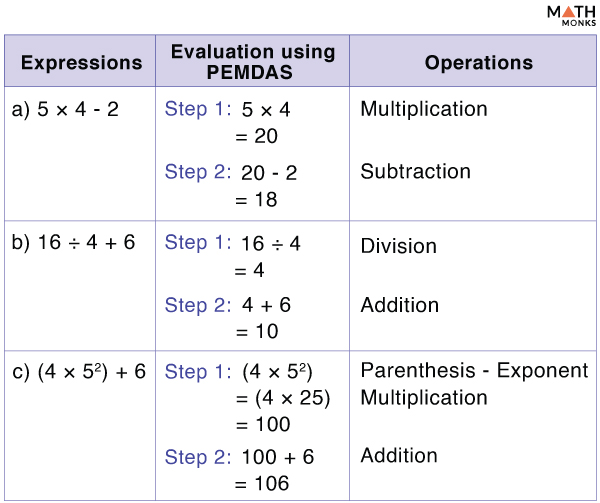Evaluate (4 × 2 ÷ 1) – 2

Solution: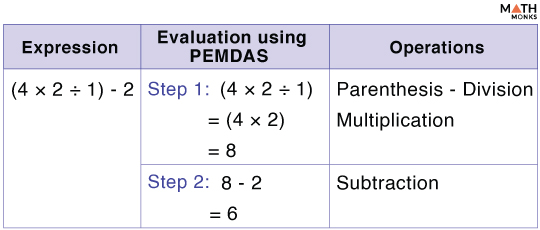Evaluate 3 + 6 x (5 + 7) ÷ 3 – 7 using PEMDAS.

Solution: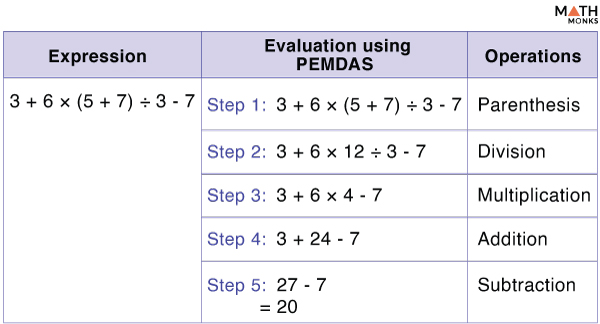Simplify the algebraic expression (8x – 2x) ÷ 3x using PEMDAS

Solution:Using the order of operation to solve the EXPRESSION with FRACTION.

Solve the given expression (5/2 × 7/2) + (7/3 × 5/3)

Solution: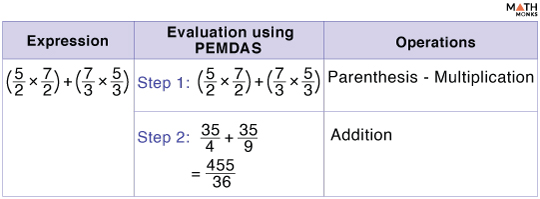Using the order of operation to solve the EXPRESSION with ABSOLUTE VALUE

Solve the given expression (-9 – (-3) x 7) ÷ |-32 + 5|

Solution: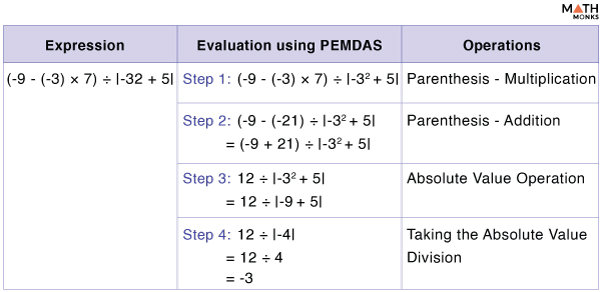## Why is the Order of Operations Important

It is essential because it offers a universal rule we can all follow to perform an expression and get the same result.

We use PEMDAS whenever we need to solve an everyday problem. Let us consider a simple word problem.

Suppose we bought 6 pieces of pizzas costing $15 each. If we distribute the 6 slices among 6 of your friends equally, each will get one piece. If we intend to find the cost of each piece, we apply PEMDAS to solve the expression. Total number of people = 6 Number of pizza slices = 6 Cost of each slice =$15

The expression becomes (15 + 15 + 15 + 15 + 15 +15) ÷ 6 or (6 × 15) ÷ 6

According to PEMDAS,

(6 × 15) ÷ 6

Similarly, we use PEMDAS to solve many similar problems in our everyday life.

Kris bought 6 bananas for 10 cents each and 1 apple for 60 cents. Write a numerical expression to represent this situation and then find the total cost in the dollar.

Solution:

According to the problem, the expression is
6 × 10 + 2 × 60
Solving the expression using PEMDAS
= 60 + 120 (Multiplication)